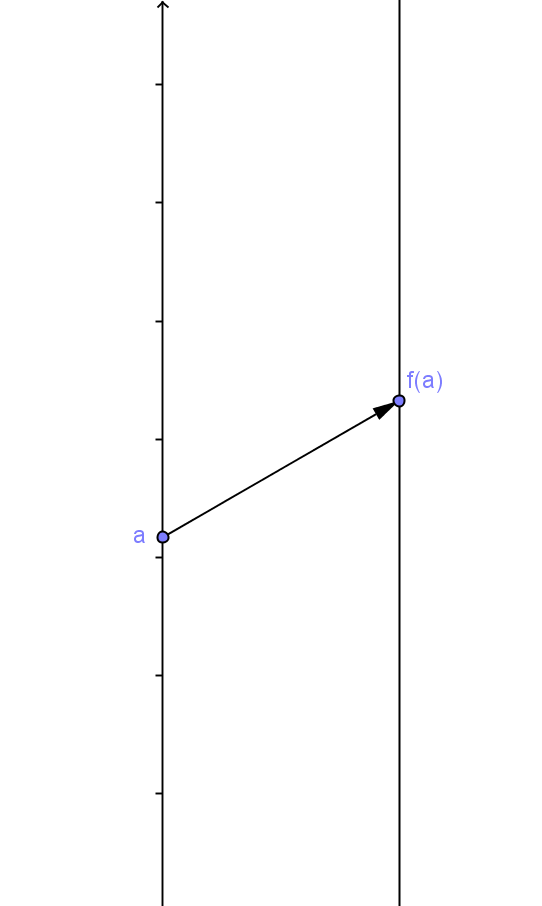Suppose \$f\$ is a real valued function with domain a subset of  real numbers, \$D\$.

Definition: A mapping diagram for \$f\$
is a figure consisting of two parallel number lines (or axes) and a set of arrows.
Points on one (source or input)  line represent numbers from the domain, the source (controlling or independent) variable values.
Points on the other (target or output) line represent numbers from the co-domain, the target (controlled or dependent) variable values.
An arrow in the diagram has its tail on a point representing a selected  number, \$a\$, on the source (domain) line.
The head of  the arrow points to the function value, \$f(a)\$,  for the number \$a\$, represented by a point on the target (co-domain) line.Conventions: When the axes are displayed vertically, the axis on the left represents the source line ( "the X axis") and the axis on the right represents the target line ("the Y axis").
Both lines are oriented with the larger numbers higher on the axes.

When the axes are displayed horizontally, the axis on the top represents the source line ( "the X axis") and the axis on the bottom represents the target line( "the Y axis").
Both lines are oriented with the larger numbers further to the right.

In this resource, mapping diagrams will be displayed vertically.